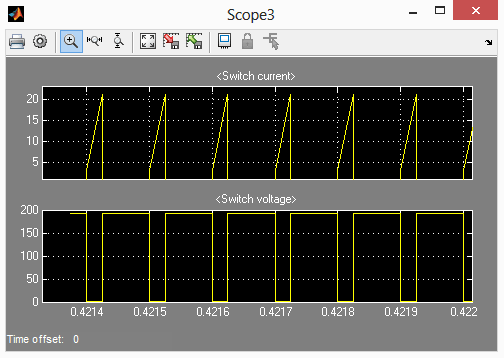design a Buck converter in Matlab | 12CAD.com

# How to design a Buck converter in Matlab

A Buck Converter is a DC – DC step down voltage converter (can also be considered like step up current converter) .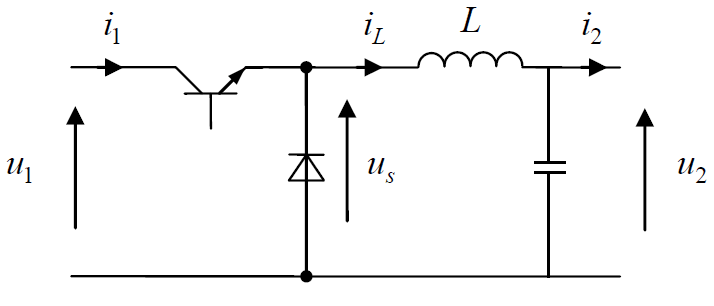Here is how you can use Matlab to simulate a Buck converter (Note: In this post, we will consider switches as ideal)

In order to get this task done in Matlab we will need the following component from Simulink Library.

Design a Buck converter in Matlab

## Main elements

### DC voltage Source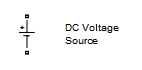Amplitude voltage: 190 V

### A Pulse Generator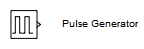Amplitude: 1

Period (sec): 0.0001

Pulse width (% of period): 25

Phase delay (secs):0

Interpret vector parameters as 1-D: activated

### An Ideal switch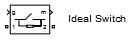Internal resistance Ron (Ohms): 0.001

Initial  state (0 to ‘open, 1 for ‘closed'): 0

Snubber resistance Rs (ohms): 1e5

Snubber capacitance Cs (F): inf

Show measurment port: activated

### A Diode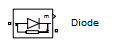Resistance Ron (ohms): 1e5

Inductance Lon (H): 0

Forward voltage Vf (V): 0.8

Initial current Ic (A): 0

Snubber resistance Rs (ohms): 500

Snubber capacitance Cs (F): 250e-9

Show measurement port: activated

### An Inductor, a Capacitor and a ResistorL=200e-6

C=300e-6

(All these 3 components are being added by using the element below, you just need to choose one or more to be included in your block/element)

In order to visualize the behavior of different voltages and currents in the circuit, we will be using the following elements.

### Current measurement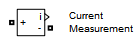### Voltage measurement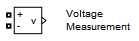### Scope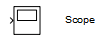Parameters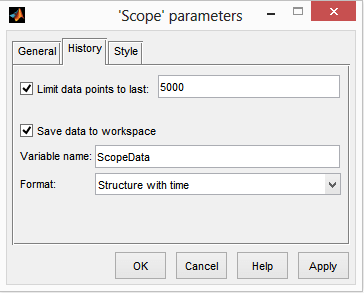### Bus Selector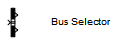Parameters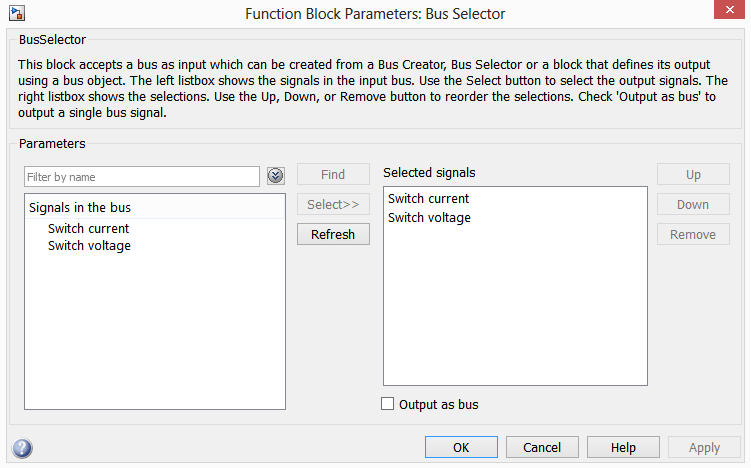### Powergui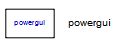Parameters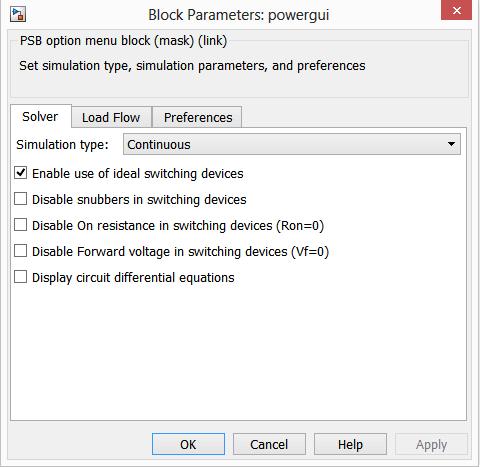## Opening Simulink Library and creating a new model in Matlab

By clicking on the icon shown on the picture below, you can open Simulink library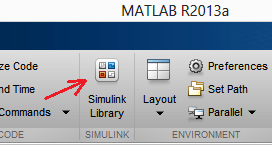The following should pop up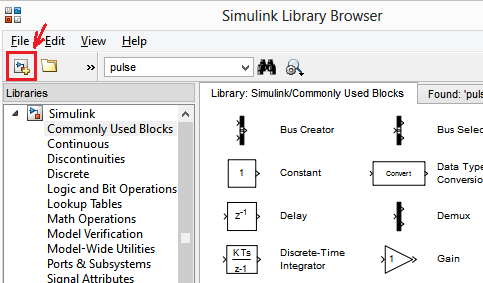You can then easily create a new model with a click on the New Model icon shown on the image above.

After opening the new model, draw the followingMost of the element are in SimScape/SimPowerSystems and in Simulink Library. You can use the research bar to find element in the library as well.

Run the model, and check different wave shapes on each on the scopes.

My output voltage shape looks like this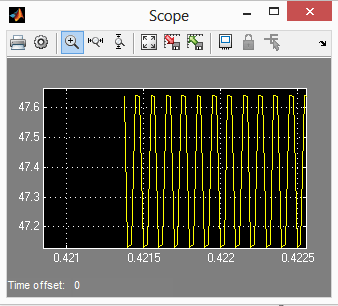Switch Current and voltage